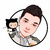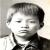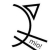## JDK中Arrays.sort 枢轴划分问题？

if array.length > 40

left = mid(xx) //left mid 和 right分别均匀的在9个位置上区得了分别的中值 为什么呢？

right = mid(xx)//我知道原因是在n非常大的时候，可以尽可能的避免最坏情况的出现，可是，怎                      //么分析出来的呢？

1.   /**
2.     * 将指定范围的整形数组升序排序。
3.     * x[] 待排数组
4.     * off 从数组的第off个元素开始排序
5.     * len 数组长度
6.     */
7.    private static void sort1(int x[], int off, int len) {
8. //优化1：在小规模(size<7)数组中，直接插入排序的效率要比快速排序高。
9. if (len < 7) {
10.     for (int i=off; i<len+off; i++)
11.         for (int j=i; j>off && x[j-1]>x[j]; j--)
12.             swap(x, j, j-1);
13.     return;
14. }
15.
16. //优化2：精心选择划分元素，即枢轴
17. //如果是小规模数组(size<=7)，直接取中间元素作为枢轴
18. //如果是中等规模数组(7=<size<=40)，则在数组首、中、尾三个位置上的数中取中间大小的数作为枢轴
19. //如果是大规模数组(size>40),则在9个指定的数中取一个伪中数(中间大小的数s)
20. int m = off + (len >> 1);
21. if (len > 7) {
22.     int l = off;
23.     int n = off + len - 1;
24.     if (len > 40) {
25.         int s = len/8;
26.         l = med3(x, l, l+s, l+2*s);
27.         m = med3(x, m-s,   m,   m+s);
28.         n = med3(x, n-2*s, n-s, n);
29.     }
30.     m = med3(x, l, m, n);
31. }
32. int v = x[m];
33.
34.         //优化3：每一次枢轴v的划分，都会形成形成一个形如  (<v)* v* (>v)*
35.        //阶段一，形成 v* (<v)* (>v)* v* 的数组
36.        int a = off, b = a, c = off + len - 1, d = c;
37.        while(true) {
38.     while (b <= c && x[b] <= v) {
39.         if (x[b] == v)
40.             swap(x, a++, b);
41.         b++;
42.     }
43.     while (c >= b && x[c] >= v) {
44.         if (x[c] == v)
45.             swap(x, c, d--);
46.         c--;
47.     }
48.     if (b > c)
49.         break;
50.     swap(x, b++, c--);
51. }
52.
53. //阶段二，将枢轴和与枢轴相等的元素交换到数组中间
54. int s, n = off + len;
55. s = Math.min(a-off, b-a  );  vecswap(x, off, b-s, s);
56. s = Math.min(d-c,   n-d-1);  vecswap(x, b,   n-s, s);
57.
58. //阶段三，递归排序与枢轴不相等都元素区间
59. if ((s = b-a) > 1)
60.     sort1(x, off, s);
61. if ((s = d-c) > 1)
62.     sort1(x, n-s, s);
63.    }

0JDK里面的划分情况，因该算是随机划分（因为原来的排序是随机的，那么选择任何一个位置，其实都是随机的），取中值，也就保证了所选择的数据对不可能是剩下的所有元素中最大或者最小的数，起码他左边和右边就有一个

00@中山野鬼 ...在少数极限情况下 处理以后的概率跟处理之前的概率 不是一个数量级的。。。 而且改变其实并不大 仅仅是对于值的选择做了一点点改动00
d

API上有说明，算法是从Jon L. Bentley（写编程珠玑那牛人）和M. Douglas McIlroy的论文 Engineering a Sort Function （93年）中得来的，论文中讨论了为什么要这样设计快排，有兴趣可以自己读一下这篇论文（在google里一搜就搜到）。

d